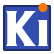KiCad Pcbnew Python Scripting
pcbnew.EDA_RECT Class Reference
Inheritance diagram for pcbnew.EDA_RECT: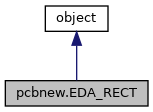[legend]

## Public Member Functions

def __init__ (self, *args)

"wxPoint" Centre (self)

"void" Move (self, "wxPoint" aMoveVector)

"void" Normalize (self)

"bool" Contains (self, *args)

"wxSize const" GetSize (self)

"int" GetSizeMax (self)

"int" GetX (self)

"int" GetY (self)

"wxPoint const" GetOrigin (self)

"wxPoint const" GetPosition (self)

"wxPoint const" GetEnd (self)

"wxPoint const" GetCenter (self)

"int" GetWidth (self)

"int" GetHeight (self)

"int" GetRight (self)

"int" GetLeft (self)

"int" GetTop (self)

"int" GetBottom (self)

"bool" IsValid (self)

"void" SetOrigin (self, *args)

"void" SetSize (self, *args)

"void" Offset (self, *args)

"void" SetX (self, "int" val)

"void" SetY (self, "int" val)

"void" SetWidth (self, "int" val)

"void" SetHeight (self, "int" val)

"void" SetEnd (self, *args)

"void" RevertYAxis (self)

"bool" Intersects (self, *args)

"wxPoint const" ClosestPointTo (self, "wxPoint" aPoint)

"wxPoint const" FarthestPointTo (self, "wxPoint" aPoint)

"bool" IntersectsCircle (self, "wxPoint" aCenter, "int const" aRadius)

"bool" IntersectsCircleEdge (self, "wxPoint" aCenter, "int const" aRadius, "int const" aWidth)

"wxRect" getWxRect (self)

"BOX2I" getBOX2I (self)

"EDA_RECT &" Inflate (self, *args)

"void" Merge (self, *args)

"double" GetArea (self)

"EDA_RECT" Common (self, "EDA_RECT" aRect)

"EDA_RECT const" GetBoundingBoxRotated (self, "wxPoint" aRotCenter, "double" aAngle)

## Properties

thisown = property(lambda x: x.this.own(), lambda x, v: x.this.own(v), doc="The membership flag")

## Detailed Description

`Proxy of C++ EDA_RECT class.`

Definition at line 1634 of file pcbnew.py.

## ◆ __init__()

 def pcbnew.EDA_RECT.__init__ ( self, * args )
```    __init__(EDA_RECT self) -> EDA_RECT
__init__(EDA_RECT self, wxPoint aPos, wxSize aSize) -> EDA_RECT```

Definition at line 1640 of file pcbnew.py.

Here is the caller graph for this function: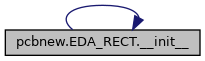## ◆ Centre()

 "wxPoint" pcbnew.EDA_RECT.Centre ( self )
`Centre(EDA_RECT self) -> wxPoint`

Definition at line 1648 of file pcbnew.py.

## ◆ ClosestPointTo()

 "wxPoint const" pcbnew.EDA_RECT.ClosestPointTo ( self, "wxPoint" aPoint )
`ClosestPointTo(EDA_RECT self, wxPoint aPoint) -> wxPoint`

Definition at line 1785 of file pcbnew.py.

## ◆ Common()

 "EDA_RECT" pcbnew.EDA_RECT.Common ( self, "EDA_RECT" aRect )
`Common(EDA_RECT self, EDA_RECT aRect) -> EDA_RECT`

Definition at line 1827 of file pcbnew.py.

## ◆ Contains()

 "bool" pcbnew.EDA_RECT.Contains ( self, * args )
```    Contains(EDA_RECT self, wxPoint aPoint) -> bool
Contains(EDA_RECT self, int x, int y) -> bool
Contains(EDA_RECT self, EDA_RECT aRect) -> bool```

Definition at line 1660 of file pcbnew.py.

Here is the caller graph for this function: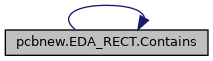## ◆ FarthestPointTo()

 "wxPoint const" pcbnew.EDA_RECT.FarthestPointTo ( self, "wxPoint" aPoint )
`FarthestPointTo(EDA_RECT self, wxPoint aPoint) -> wxPoint`

Definition at line 1789 of file pcbnew.py.

## ◆ GetArea()

 "double" pcbnew.EDA_RECT.GetArea ( self )
`GetArea(EDA_RECT self) -> double`

Definition at line 1823 of file pcbnew.py.

## ◆ GetBottom()

 "int" pcbnew.EDA_RECT.GetBottom ( self )
`GetBottom(EDA_RECT self) -> int`

Definition at line 1720 of file pcbnew.py.

## ◆ GetBoundingBoxRotated()

 "EDA_RECT const" pcbnew.EDA_RECT.GetBoundingBoxRotated ( self, "wxPoint" aRotCenter, "double" aAngle )
`GetBoundingBoxRotated(EDA_RECT self, wxPoint aRotCenter, double aAngle) -> EDA_RECT`

Definition at line 1831 of file pcbnew.py.

## ◆ getBOX2I()

 "BOX2I" pcbnew.EDA_RECT.getBOX2I ( self )
`getBOX2I(EDA_RECT self) -> BOX2I`

Definition at line 1805 of file pcbnew.py.

## ◆ GetCenter()

 "wxPoint const" pcbnew.EDA_RECT.GetCenter ( self )
`GetCenter(EDA_RECT self) -> wxPoint`

Definition at line 1696 of file pcbnew.py.

## ◆ GetEnd()

 "wxPoint const" pcbnew.EDA_RECT.GetEnd ( self )
`GetEnd(EDA_RECT self) -> wxPoint`

Definition at line 1692 of file pcbnew.py.

## ◆ GetHeight()

 "int" pcbnew.EDA_RECT.GetHeight ( self )
`GetHeight(EDA_RECT self) -> int`

Definition at line 1704 of file pcbnew.py.

Here is the caller graph for this function: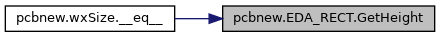## ◆ GetLeft()

 "int" pcbnew.EDA_RECT.GetLeft ( self )
`GetLeft(EDA_RECT self) -> int`

Definition at line 1712 of file pcbnew.py.

## ◆ GetOrigin()

 "wxPoint const" pcbnew.EDA_RECT.GetOrigin ( self )
`GetOrigin(EDA_RECT self) -> wxPoint`

Definition at line 1684 of file pcbnew.py.

## ◆ GetPosition()

 "wxPoint const" pcbnew.EDA_RECT.GetPosition ( self )
`GetPosition(EDA_RECT self) -> wxPoint`

Definition at line 1688 of file pcbnew.py.

## ◆ GetRight()

 "int" pcbnew.EDA_RECT.GetRight ( self )
`GetRight(EDA_RECT self) -> int`

Definition at line 1708 of file pcbnew.py.

## ◆ GetSize()

 "wxSize const" pcbnew.EDA_RECT.GetSize ( self )
`GetSize(EDA_RECT self) -> wxSize`

Definition at line 1668 of file pcbnew.py.

## ◆ GetSizeMax()

 "int" pcbnew.EDA_RECT.GetSizeMax ( self )
`GetSizeMax(EDA_RECT self) -> int`

Definition at line 1672 of file pcbnew.py.

## ◆ GetTop()

 "int" pcbnew.EDA_RECT.GetTop ( self )
`GetTop(EDA_RECT self) -> int`

Definition at line 1716 of file pcbnew.py.

## ◆ GetWidth()

 "int" pcbnew.EDA_RECT.GetWidth ( self )
`GetWidth(EDA_RECT self) -> int`

Definition at line 1700 of file pcbnew.py.

Here is the caller graph for this function:## ◆ getWxRect()

 "wxRect" pcbnew.EDA_RECT.getWxRect ( self )
`getWxRect(EDA_RECT self) -> wxRect`

Definition at line 1801 of file pcbnew.py.

## ◆ GetX()

 "int" pcbnew.EDA_RECT.GetX ( self )
`GetX(EDA_RECT self) -> int`

Definition at line 1676 of file pcbnew.py.

## ◆ GetY()

 "int" pcbnew.EDA_RECT.GetY ( self )
`GetY(EDA_RECT self) -> int`

Definition at line 1680 of file pcbnew.py.

## ◆ Inflate()

 "EDA_RECT &" pcbnew.EDA_RECT.Inflate ( self, * args )
```    Inflate(EDA_RECT self, wxCoord dx, wxCoord dy) -> EDA_RECT
Inflate(EDA_RECT self, int aDelta) -> EDA_RECT```

Definition at line 1809 of file pcbnew.py.

Here is the caller graph for this function: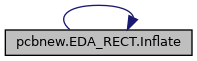## ◆ Intersects()

 "bool" pcbnew.EDA_RECT.Intersects ( self, * args )
```    Intersects(EDA_RECT self, EDA_RECT aRect) -> bool
Intersects(EDA_RECT self, EDA_RECT aRect, double aRot) -> bool
Intersects(EDA_RECT self, wxPoint aPoint1, wxPoint aPoint2) -> bool
Intersects(EDA_RECT self, wxPoint aPoint1, wxPoint aPoint2, wxPoint aIntersection1, wxPoint aIntersection2) -> bool```

Definition at line 1776 of file pcbnew.py.

Here is the caller graph for this function: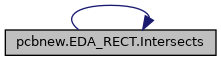## ◆ IntersectsCircle()

 "bool" pcbnew.EDA_RECT.IntersectsCircle ( self, "wxPoint" aCenter, "int const" aRadius )
`IntersectsCircle(EDA_RECT self, wxPoint aCenter, int const aRadius) -> bool`

Definition at line 1793 of file pcbnew.py.

## ◆ IntersectsCircleEdge()

 "bool" pcbnew.EDA_RECT.IntersectsCircleEdge ( self, "wxPoint" aCenter, "int const" aRadius, "int const" aWidth )
`IntersectsCircleEdge(EDA_RECT self, wxPoint aCenter, int const aRadius, int const aWidth) -> bool`

Definition at line 1797 of file pcbnew.py.

## ◆ IsValid()

 "bool" pcbnew.EDA_RECT.IsValid ( self )
`IsValid(EDA_RECT self) -> bool`

Definition at line 1724 of file pcbnew.py.

## ◆ Merge()

 "void" pcbnew.EDA_RECT.Merge ( self, * args )
```    Merge(EDA_RECT self, EDA_RECT aRect)
Merge(EDA_RECT self, wxPoint aPoint)```

Definition at line 1816 of file pcbnew.py.

Here is the caller graph for this function: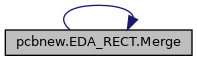## ◆ Move()

 "void" pcbnew.EDA_RECT.Move ( self, "wxPoint" aMoveVector )
`Move(EDA_RECT self, wxPoint aMoveVector)`

Definition at line 1652 of file pcbnew.py.

## ◆ Normalize()

 "void" pcbnew.EDA_RECT.Normalize ( self )
`Normalize(EDA_RECT self)`

Definition at line 1656 of file pcbnew.py.

## ◆ Offset()

 "void" pcbnew.EDA_RECT.Offset ( self, * args )
```    Offset(EDA_RECT self, int dx, int dy)
Offset(EDA_RECT self, wxPoint offset)```

Definition at line 1742 of file pcbnew.py.

Here is the caller graph for this function:## ◆ RevertYAxis()

 "void" pcbnew.EDA_RECT.RevertYAxis ( self )
`RevertYAxis(EDA_RECT self)`

Definition at line 1772 of file pcbnew.py.

## ◆ SetEnd()

 "void" pcbnew.EDA_RECT.SetEnd ( self, * args )
```    SetEnd(EDA_RECT self, int x, int y)
SetEnd(EDA_RECT self, wxPoint pos)```

Definition at line 1765 of file pcbnew.py.

Here is the caller graph for this function: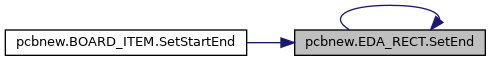## ◆ SetHeight()

 "void" pcbnew.EDA_RECT.SetHeight ( self, "int" val )
`SetHeight(EDA_RECT self, int val)`

Definition at line 1761 of file pcbnew.py.

Here is the caller graph for this function: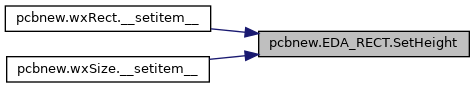## ◆ SetOrigin()

 "void" pcbnew.EDA_RECT.SetOrigin ( self, * args )
```    SetOrigin(EDA_RECT self, wxPoint pos)
SetOrigin(EDA_RECT self, int x, int y)```

Definition at line 1728 of file pcbnew.py.

Here is the caller graph for this function: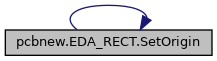## ◆ SetSize()

 "void" pcbnew.EDA_RECT.SetSize ( self, * args )
```    SetSize(EDA_RECT self, wxSize size)
SetSize(EDA_RECT self, int w, int h)```

Definition at line 1735 of file pcbnew.py.

Here is the caller graph for this function: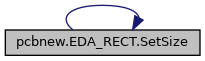## ◆ SetWidth()

 "void" pcbnew.EDA_RECT.SetWidth ( self, "int" val )
`SetWidth(EDA_RECT self, int val)`

Definition at line 1757 of file pcbnew.py.

Here is the caller graph for this function: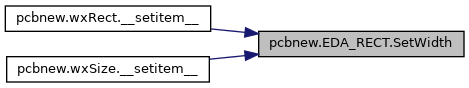## ◆ SetX()

 "void" pcbnew.EDA_RECT.SetX ( self, "int" val )
`SetX(EDA_RECT self, int val)`

Definition at line 1749 of file pcbnew.py.

Here is the caller graph for this function:## ◆ SetY()

 "void" pcbnew.EDA_RECT.SetY ( self, "int" val )
`SetY(EDA_RECT self, int val)`

Definition at line 1753 of file pcbnew.py.

Here is the caller graph for this function: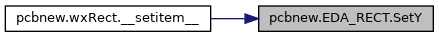## ◆ thisown

 pcbnew.EDA_RECT.thisown = property(lambda x: x.this.own(), lambda x, v: x.this.own(v), doc="The membership flag")
static

Definition at line 1637 of file pcbnew.py.

The documentation for this class was generated from the following file: